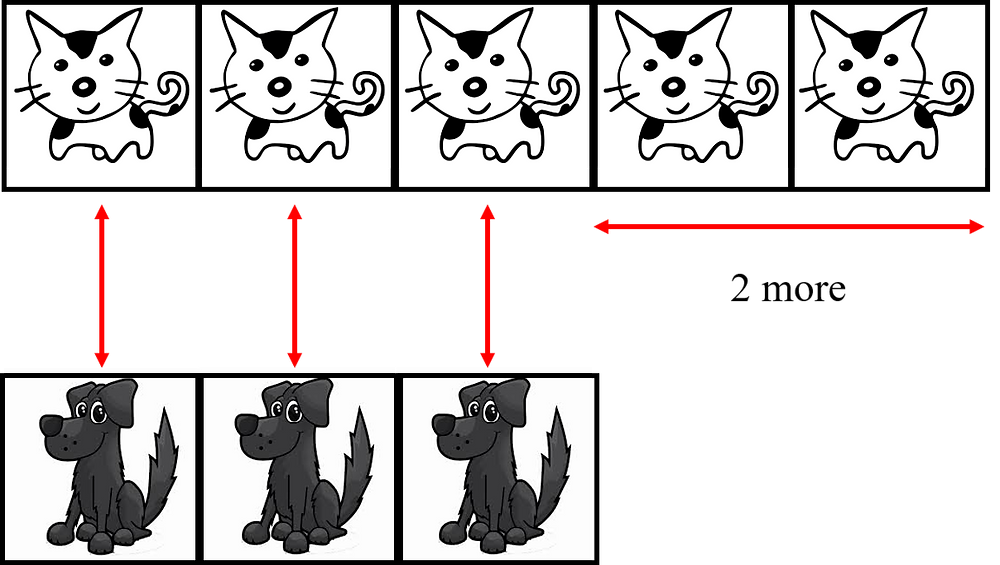Search

# What is Comparison Model?

In the previous posts, I have shared on one of the two basic types of model drawing: Part-Whole. In this post, I will be continuing on the next model type: Comparison Model.

Question

Mary has 5 cats and 3 dogs in her house. How many more cats than dogs does Mary have?

Concrete Method

Students can count the number of objects or pictures of cats and dogs as shown below and match one cat with one dog. After matching, there will be 2 cats left. This instinctively shows students that there are 2 more cats than dogs.This is how students can match objects and count the difference of the two objects. This is the Concrete aspect.

Pictorial Method

After students are comfortable with physically matching and counting the difference, they may be guided to the pictorial method: Model Drawing.

First, students can classify each object in a small block as shown below so they understand that each identical box contains one object. So the difference is also 2 boxes.Next, the objects in the respective boxes can be removed to allow students to transit to the pictorial. At this stage students should still have the understanding that each box is identical and each box contains one object. In this manner, students still manage to see that there are 2 extra boxes.After which, students may be guided to draw boxes with varying lengths. The longer the length of the boxes, the larger the quantity it represents. In the diagram below, it should be highlighted that:

• boxes representing 3 cats and 3 dogs respectively should have the same length

• the box that represents 2 more cats represents the difference between the two quantities

The term "difference" should also be introduced while dealing with Comparison Model.At last, students can be brought to the last diagram where this is where students would need to draw while reading the word problem. They should draw the larger quantity longer than the other quantity. The shortfall or the space between the two models is known as the difference.This is the Pictorial Model Method. With such systematic step by step, we can guide our children in drawing Comparison Model as shown and this helps them in comprehending the word problem as they start to draw such basic models.

Abstract Method

Essentially, you want your child to be able to write 5 - 3 = 2. However, he or she may not appreciate why you need to subtract (-) the two numbers and what does the '2' represent. Hence, the pictorial model allows your child to instinctively come up with the solution and get your child to explain to you why he or she chose to subtract. This will in turn allow your child to learn the Mathematical thinking by himself or herself and build conceptual understanding.Example of how Comparison Model looks like in a word problem.

Taken from PSLE Prep Programme.

Summary

Comparison Model is used when you are required to compare two or more quantities and find the difference.

The difference is the quantity between the larger and smaller quantities.

Difference = Larger quantity - Smaller quantity

Smaller quantity + Difference = Larger Quantity

That's it! This is the Comparison Model and do practise this model type with your child! We have learnt the two basic types of Singapore Model Method: Part-Whole Model and Comparison Model. Before moving on to subsequent model drawing, do take the time to review these two basic type as both of them forms the backbone of the other model types.

In the next few articles, I will be sharing on how to use the Part-Whole Model and Comparison Model to learn and consolidate the concept of Arithmetic.